Learning Library

# Fraction Action

Get your kid in on the fraction action! She'll get great fraction practice with these worksheets on dividing, multiplying, adding and subtracting fractions.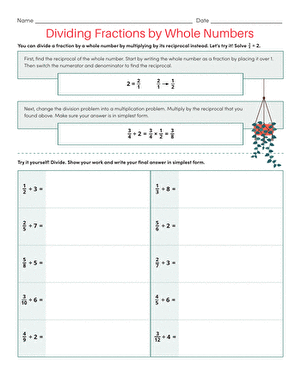### Dividing Fractions with Whole Numbers

Challenge your child to practice dividing fractions with whole numbers on this clear and simple worksheet.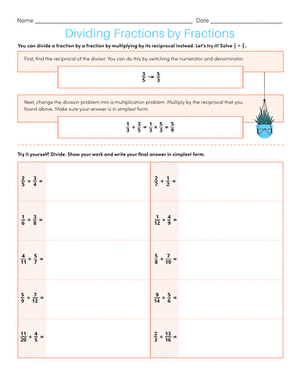### Dividing Fractions by Fractions

Your child will learn how to divide a fraction with a fraction on this simple, step-by-step worksheet.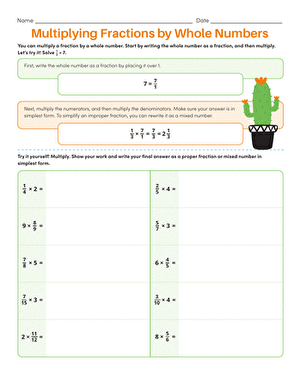### Multiplying Fractions with Whole Numbers

Use this worksheet to give your child practice multiplying fractions with whole numbers.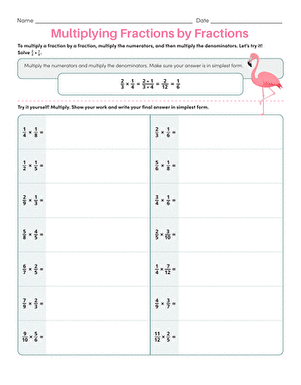### Multiplying Fractions with Fractions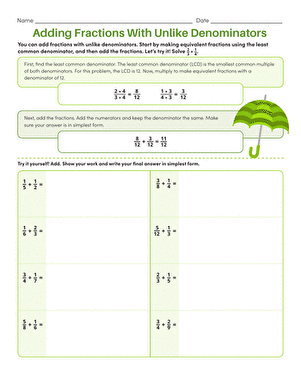### Adding Fractions with Unlike Denominators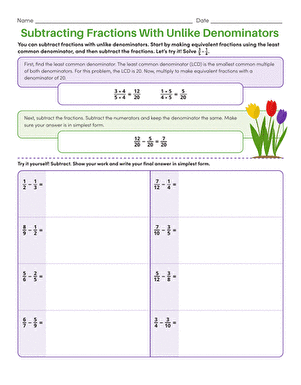### Subtracting Fractions with Unlike Denominators

Help your child understand this process of subtracting fractions with unlike denominators through using this simple worksheet.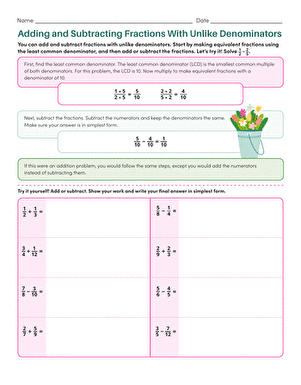### Adding and Subtracting with Unlike Denominators

This worksheet is sure to help any child that needs practice adding and subtracting fractions with unlike denominators.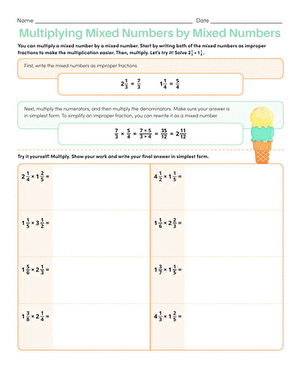### How to Multiply Mixed Numbers by Mixed Numbers

This valuable worksheet teaches your little mathematician how to convert mixed numbers to fractions, and then multiply them.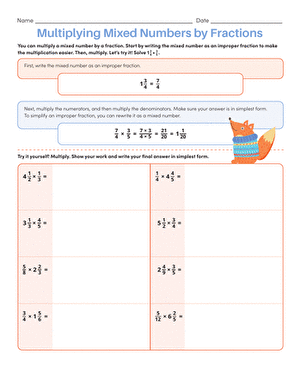### Multiply Mixed Numbers with Fractions

This colorful worksheet shows your child how to multiply a mixed number with a fraction, and gives him practice with the method.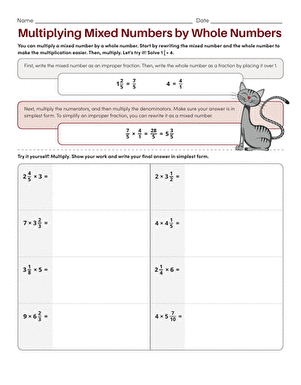### Multiply Mixed Numbers with Whole Numbers

Multiplying mixed numbers and whole numbers: it looks rather complicated, but it's actually a matter of learning just a few steps.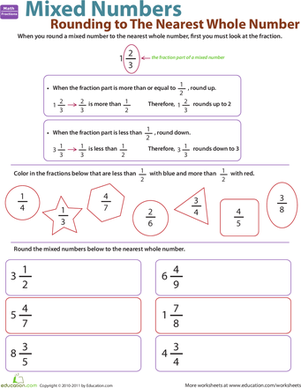### Rounding to the Nearest Whole Number

Does your little mathematician know how to round mixed numbers up or down? This worksheet shows her how.

Create new collection

0

### New Collection>

0 items

What could we do to improve Education.com?